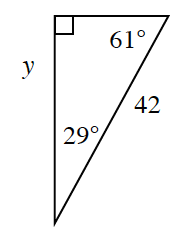### Home > CCG > Chapter 5 > Lesson 5.3.3 > Problem5-100

5-100.Eugene wants to use the cosine ratio to find $y$ on this triangle.

1. Which angle should he use to write an equation and solve for $y$ using the cosine ratio? Why?

He'll need the adjacent side and the hypotenuse. To which angle is $y$ adjacent?

2. Set up an equation, and solve for $y$ using cosine.

$\cos29º=\frac{y}{42}$